Select Page

In a previous couple of articles, we explored some basic machine learning algorithms. Thus far we covered some simple regression algorithms, classification algorithms. We used ML.NET implementation and application of these algorithms. Up to this point, we explored algorithms that are using supervised learning. This means that we always had input and expected output data that we used to train our machine learning models. In this type of learning, the training set contains inputs and desired outputs. This way the algorithm can check its calculated output the same as the desired output and take appropriate actions based on that.

Are you afraid that AI might take your job? Make sure you are the one who is building it.

STAY RELEVANT IN THE RISING AI INDUSTRY! 🖖

## 1. Clustering Intuition

However, in real life, we often don’t have both input and output data, but we only have input data. This means that the algorithm on itself needs to figure connections between input samples. For that, we use unsupervised learning. In unsupervised learning, the training set contains only inputs. Just like we solve regression and classification problems with supervised learning with unsupervised learning we solve clustering problems. This technique attempts to identify similar inputs and to put them into categories, ie. it clusters data. Generally speaking, the goal is to detect the hidden patterns among the data and group them into clusters. This means that the samples which have some shared properties will fall into one group – cluster.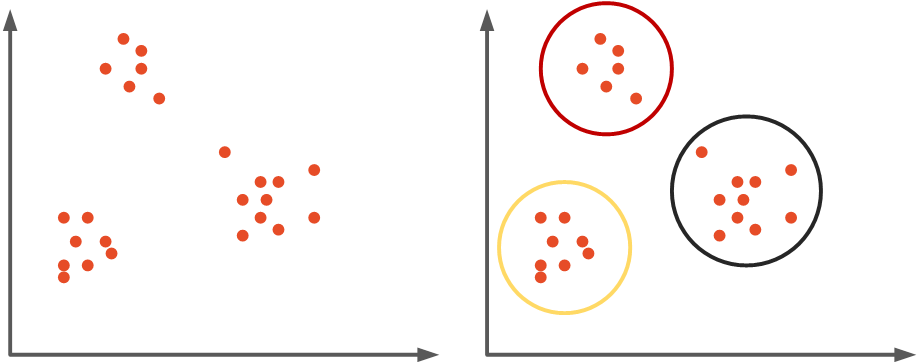There are many clustering algorithms out there and in this article, and in this article we focus on K-Means Clustering because this algorithm is supported in ML.NET. However, we will explore a bit other types of clustering like Agglomerative Clustering and DBSCAN, but the focus remains on K-Means.

## 2. Dataset and Prerequisites

Data that we use in this article is from PalmerPenguins Dataset. This dataset has been recently introduced as an alternative to the famous Iris dataset. It is created by Dr. Kristen Gorman and the Palmer Station, Antarctica LTER. You can obtain this dataset here, or via Kaggle. This dataset is essentially composed of two datasets, each containing data of 344 penguins. Just like in Iris dataset there are 3 different species of penguins coming from 3 islands in the Palmer Archipelago. Also, these datasets contain culmen dimensions for each species. The culmen is the upper ridge of a bird’s bill. In the simplified penguin’s data, culmen length and depth are renamed as variables culmen_length_mm and culmen_depth_mm.Data itself is not too complicated. In essence, it is just tabular data: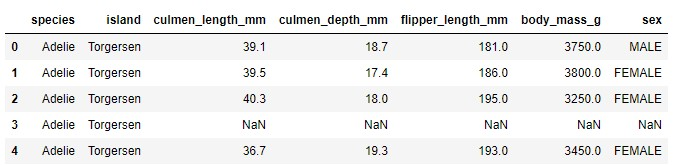Note that in this tutorial, we ignore the species feature. This is because we perform unsupervised learning, ie. we don’t need the expected output value of the sample. We want our algorithm to figure that out on its own. Here is how data looks like when we plot it: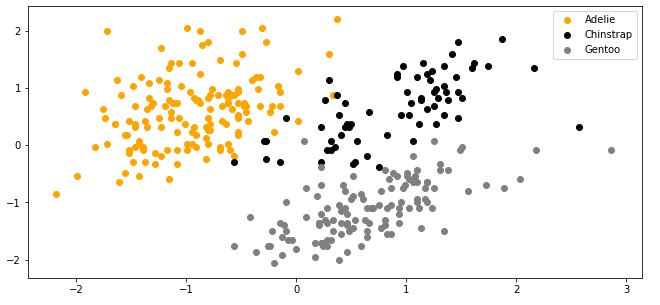The implementations provided here are done in C#, and we use the latest .NET 5. So make sure that you have installed this SDK. If you are using Visual Studio this comes with version 16.8.3. Also, make sure that you have installed the following package:

``Install-Package Microsoft.ML``

You can do a similar thing using Visual Studio’s Manage NuGetPackage option: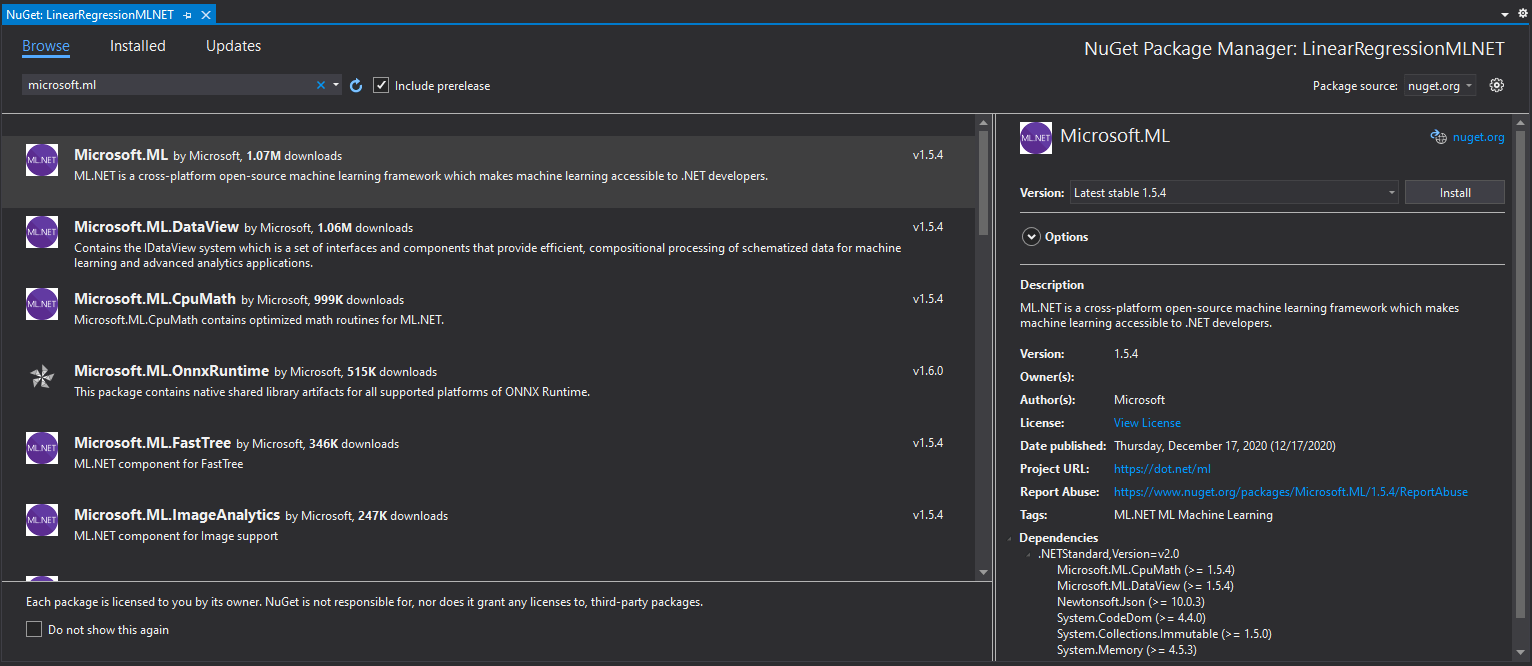If you need to catch up with the basics of machine learning with ML.NET check out this article

## 3. K-Means Clustering

K-Means is one of the most popular clustering algorithms. It is definitely a go-to option when you start experimenting with your unlabeled data. This algorithm groups n data points into K number of clusters, as the name of the algorithm suggests. This algorithm can be split into several stages:

1. In the first stage, we need to set the hyperparameter k. This represents the number of clusters or groups that K-Means Clustering will create once it is done.
2. K random vectors are picked in the feature space. These vectors are called centroids. These vectors are changed during the training process and the goal is to put them into the “center” of each cluster.
3. Distances from each input sample x to each centroid c is calculated using some metric, like Euclidean distance. The closest centroid is assigned to each sample in the dataset. Basically, we create clusters at this stage.
4. For each cluster average feature vector is calculated using samples that are assigned to it. This value is considered as a new centroid of the cluster.
5. Steps 2-4 are repeated for a fixed number of iteration or until the centroids don’t change, whichever comes first.Mathematically speaking each sample x is assigned to a cluster based on: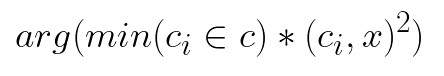where cᵢ is a centroid of a cluster i and D is Euclidean distance calculated using the formula:For finding the new centroid from clustered group of points we use formula: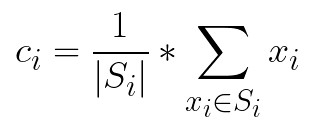As we already mentioned hyperparameter k, ie. the number of clusters, has to be tuned manually. This is quite tiresome. Later in this tutorial, we consider one technique for selecting the correct number of clusters. However, let’s explore some other types of clustering that are not yet supported in ML.NET.

## 3. Other Types of Clustering

### 3.1 Agglomerative Clustering

As we can see one of the biggest challenges of working with K-Means is that we need to determine the number of clusters beforehand. Another challenge is that K-Means tries to make clusters of the same size. These challenges can be addressed with other algorithms like Hierarchical Clustering. In general, every Hierarchical Clustering method starts by putting all samples into separate single-sample clusters. Then based on some similarity metrics, samples or clusters are merged together until the point when all samples are put into a single cluster. This means that we are building the hierarchy of clusters, hence the name. In this article, we explore Agglomerative Clustering which is one specific type of Hierarchical Clustering. The metric that it uses for merging clusters is the distance, ie. it merges the closest pair of clusters, based on the distance among centroids and repeats this step until only a single cluster is left. For this purpose, the proximity matrix is used. This matrix stores the distances between each point.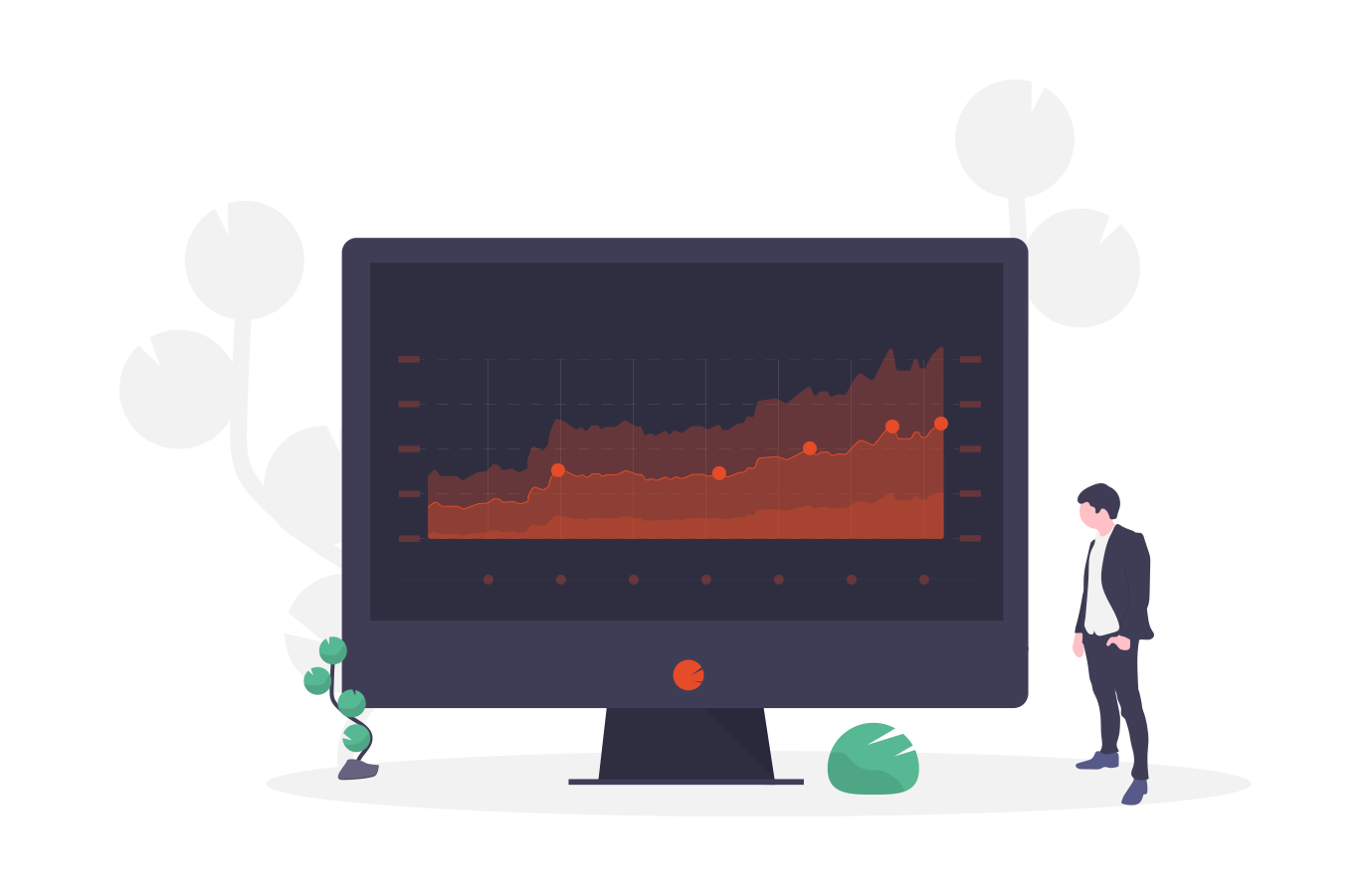Let’s break this into steps:

1. Every point is stored in its own cluster
2. The proximity Matrix is calculated
3. Closest points are detected and merged. They are the cluster and centroid is calculated.
4. The proximity matrix is updated using the centroid of the created cluster.
5. Steps 3 and 4 are repeated until one cluster is created.

At this moment you might ask yourself, how does this help us with deciding the number of clusters? To do so, we utilize an awesome concept – Dendrogram. This is a tree diagram that records all merges that happened during the training process. So, every time we merge two points or clusters, this is stored in the dendrogram. Here is one example: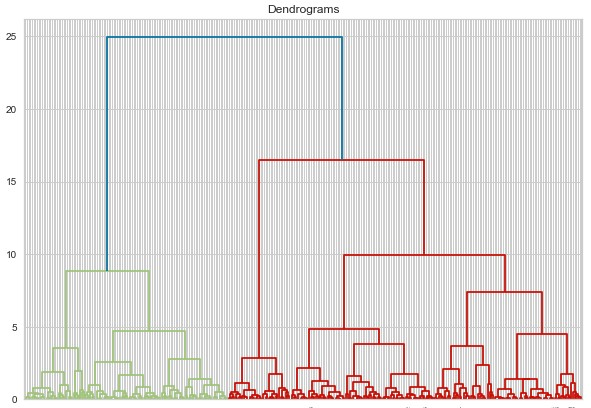What are we looking at? Well, on the x-axis we have all points in the dataset, while on the y-axis we have distances between those points. Every time points or clusters are merged this is represented with a horizontal line. The vertical line represents the distances between merged points/clusters. Longer vertical lines in the dendrogram indicate that the distance between clusters is bigger. In the next step, we need to set a threshold distance and draw a horizontal line in this image. In general, we try to set the threshold in such a way that it cuts the tallest vertical line. In our example, we set it to 15. Here is how that is done:

### 3.2 DBSCAN

Unlike K-Means and Hierarchical Clustering, which are centroid-based algorithms, DBSCAN is a density-based algorithm. Effectively, this means that you don’t need to determine how many clusters do you need. We saw this at Hierarchical clustering, but DBSCAN takes it to another level. Instead of defining hyperparameter k, we define two hyperparameters for distance ε and the number of samples per cluster – n. Let’s break it into steps and it will be more clear:

1. First, we assign a random sample x to the first cluster.
2. We count how many samples have a distance from the sample x that is less or equal to ε. If the number of such samples is greater or equal to n, we add them in the cluster.
3. We observe each new member of the cluster and perform step 2 for them too, ie. we calculate the number of samples within the ε area of the sample and if that number is larger then n, we add them to the cluster. We repeat this process recursively until there are no more samples left to put in it.
4. Steps from 1 to 3 for a new random unclustered sample.
5. The process is repeated like this until all samples are either clustered or marked as outliers.The main advantage of this approach is that clusters have different and random shapes. Centroid-based algorithms always create clusters that have the shape of a hypersphere. That is why DBSCAN can be specifically useful for some data. Of course, the main problem is choosing optimal values for ε and n. This problem is optimized with a variation of this algorithm called HDBSCAN, ie. high-performance DBSCAN. This algorithm eliminates the use of the ε hyperparameter, however, this algorithm is out of the scope of this tutorial.

## 4. Implementation with ML.NET

As we mentioned, ML.NET supports only K-Means Clustering. However, we will make our solution in a way that we expect the guys and gals from Microsoft to provide other types of clustering. That is why our solution might look over-engineered, however, we have done it so because of future flexibility.

### 4.1 High-Level Architecutre

Before we dive into the ML.NET implementation, let’s consider the high-level architecture of this implementation. In general, we want to build a solution that we can easily extend with new clustering algorithms that ML.NET might include in the future. With that in mind we create folder structure of our solution, that looks like this: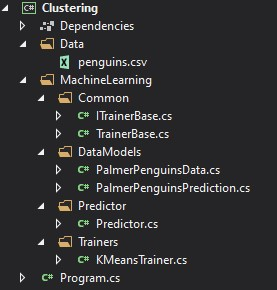The Data folder contains .csv with input data and the MachineLearning folder contains everything that is necessary for our algorithm to work. The architectural overview can be represented like this: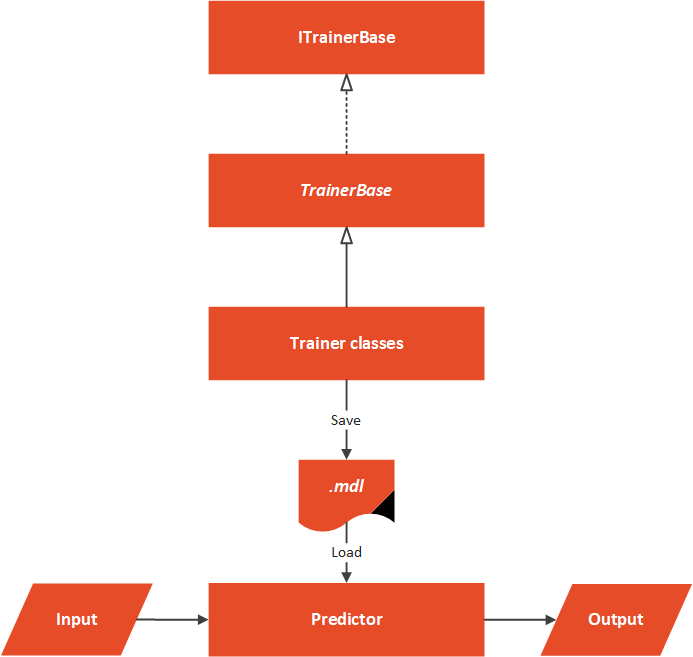At the core of this solution, we have an abstract TrainerBase class. This class is in the Common folder and its main goal is to standardize the way this whole process is done. It is in this class where we process data and perform feature engineering. This class is also in charge of training machine learning algorithms. The classes that implement this abstract class are located in the Trainers folder. In this particular case, we have only one class that does so. Here we utilize ML.NET K-Means algorithm. Once Microsoft adds new algorithms, we can extend this folder with new classes. These classes define which algorithm should be used. In this particular case, we have only one Predictor located in the Predictor folder.

### 4.2 Data Models

In order to load data from the dataset and use it with ML.NET algorithms, we need to implement classes that are going to model this data. Two files can be found in Data Folder: PalmerPenguinData and PricePalmerPenguinPredictions. The PalmerPenguinData class models input data and it looks like this:

``````using Microsoft.ML.Data;

namespace Clustering.MachineLearning.DataModels
{
/// <summary>
/// Models Palmer Penguins Binary Data.
/// </summary>
public class PalmerPenguinsData
{
public string Island { get; set; }

public float CulmenLength { get; set; }

public float CulmenDepth { get; set; }

public float FliperLength { get; set; }

public float BodyMass { get; set; }

public string Sex { get; set; }
}
}
``````

Note that we skipped loading of the first class that represents the class of the penguin. We do this because we want to perform unsupervised learning. Meaning, we don’t use the species column during the training process.The PricePalmerPenguinPredictions class models output data:

``````using Microsoft.ML.Data;

namespace Clustering.MachineLearning.DataModels
{
/// <summary>
/// Models Palmer Penguins Binary Prediction.
/// </summary>
public class PalmerPenguinsPrediction
{
[ColumnName("PredictedLabel")]
public uint PredictedClusterId;

[ColumnName("Score")]
public float[] Distances;
}
}
``````

### 4.3 TrainerBase and ITrainerBase

As we mentioned, this class is the core of this implementation. In essence, there are two parts to it. The first one is the interface that describes this class and another is the abstract class that needs to be overridden with the concrete implementations, however, it implements interface methods. Here is the ITrainerBase interface:

``````using Microsoft.ML.Data;

namespace Clustering.MachineLearning.Common
{
public interface ITrainerBase
{
string Name { get; }
void Fit(string trainingFileName);
ClusteringMetrics Evaluate();
void Save();
}
}
``````

The TrainerBase class implements this interface. However, it is abstract since we want to inject specific algorithms:

``````using Clustering.MachineLearning.DataModels;
using Microsoft.ML;
using Microsoft.ML.Calibrators;
using Microsoft.ML.Data;
using Microsoft.ML.Trainers;
using Microsoft.ML.Transforms;
using System;
using System.IO;

namespace Clustering.MachineLearning.Common

{
/// <summary>
/// Base class for Trainers.
/// This class exposes methods for training, evaluating and saving ML Models.
/// Classes that inherit this class need to assing concrete model and name.
/// </summary>
public abstract class TrainerBase<TParameters> : ITrainerBase
where TParameters : class
{
public string Name { get; protected set; }

protected static string ModelPath => Path.Combine(AppContext.BaseDirectory, "cluster.mdl");

protected DataOperationsCatalog.TrainTestData _dataSplit;
protected ITrainerEstimator<ClusteringPredictionTransformer<TParameters>, TParameters>
_model;
protected ITransformer _trainedModel;

protected TrainerBase()
{
MlContext = new MLContext(111);
}

/// <summary>
/// Train model on defined data.
/// </summary>
/// <param name="trainingFileName"></param>
public void Fit(string trainingFileName)
{
if (!File.Exists(trainingFileName))
{
throw new FileNotFoundException(\$"File {trainingFileName} doesn't exist.");
}

var dataProcessPipeline = BuildDataProcessingPipeline();
var trainingPipeline = dataProcessPipeline
.Append(_model);

_trainedModel = trainingPipeline.Fit(_dataSplit.TrainSet);
}

/// <summary>
/// Evaluate trained model.
/// </summary>
/// <returns>Metrics object which contain information about model performance.</returns>
public ClusteringMetrics Evaluate()
{
var testSetTransform = _trainedModel.Transform(_dataSplit.TestSet);

return MlContext.Clustering.Evaluate(
data: testSetTransform,
labelColumnName: "PredictedLabel",
scoreColumnName: "Score",
featureColumnName: "Features");
}

/// <summary>
/// Save Model in the file.
/// </summary>
public void Save()
{
MlContext.Model.Save(_trainedModel, _dataSplit.TrainSet.Schema, ModelPath);
}

/// <summary>
/// Feature engeneering and data pre-processing.
/// </summary>
/// <returns>Data Processing Pipeline.</returns>
private EstimatorChain<ColumnConcatenatingTransformer> BuildDataProcessingPipeline()
{
var dataProcessPipeline =
MlContext.Transforms.Text
.FeaturizeText(inputColumnName: "Sex", outputColumnName: "SexFeaturized")
.Append(MlContext.Transforms.Text
.FeaturizeText(inputColumnName: "Island", outputColumnName: "IslandFeaturized"))
.Append(MlContext.Transforms.Concatenate("Features",
"IslandFeaturized",
nameof(PalmerPenguinsData.CulmenLength),
nameof(PalmerPenguinsData.CulmenDepth),
nameof(PalmerPenguinsData.BodyMass),
nameof(PalmerPenguinsData.FliperLength),
"SexFeaturized"
))
.AppendCacheCheckpoint(MlContext);

return dataProcessPipeline;
}

{
trainingFileName,
separatorChar: ',');
return MlContext.Data.TrainTestSplit(trainingDataView, testFraction: 0.3);
}
}
}
``````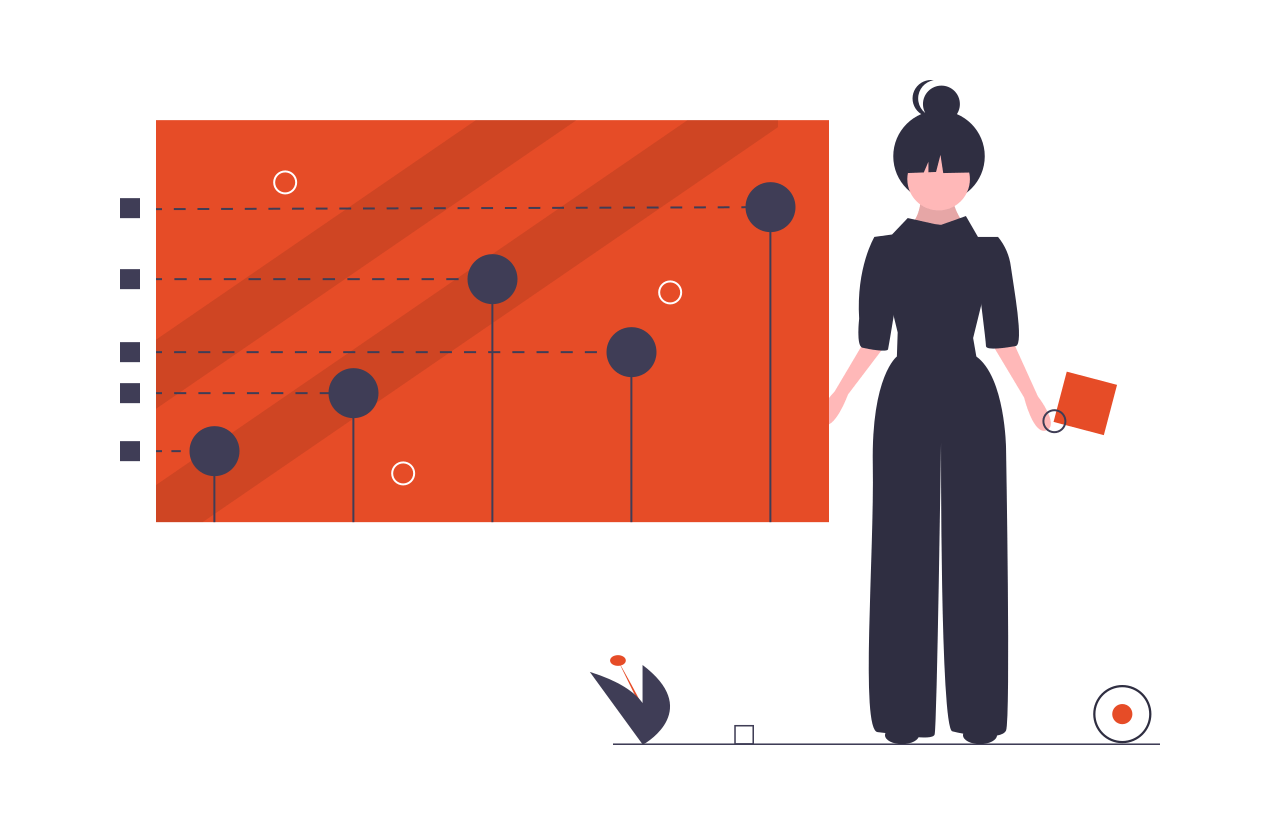That is one large class. It controls the whole process. Let’s split it up and see what it is all about. First, let’s observe the fields and properties of this class:

``````public string Name { get; protected set; }

protected static string ModelPath => Path.Combine(AppContext.BaseDirectory, "cluster.mdl");

protected DataOperationsCatalog.TrainTestData _dataSplit;
protected ITrainerEstimator<ClusteringPredictionTransformer<TParameters>, TParameters> _model;
protected ITransformer _trainedModel;``````

The Name property is used by the class that inherits this one to add the name of the algorithm. The ModelPath field is there to define where we will store our model once it is trained. Note that the file name has .mdl extension. Then we have our MlContext so we can use ML.NET functionalities. Don’t forget that this class is a singleton, so there will be only one in our solution. The _dataSplit field contains loaded data. Data is split into train and test datasets within this structure.

The field _model is used by the child classes. These classes define which machine learning algorithm is used in this field. The _trainedModel field is the resulting model that should be evaluated and saved. In essence, the only job of the class that inherits and implements this one is to define the algorithm that should be used, by instantiating an object of the desired algorithm as _model

Cool, let’s now explore Fit() method:

``````public void Fit(string trainingFileName)
{
if (!File.Exists(trainingFileName))
{
throw new FileNotFoundException(\$"File {trainingFileName} doesn't exist.");
}

var dataProcessPipeline = BuildDataProcessingPipeline();
var trainingPipeline = dataProcessPipeline.Append(_model);

_trainedModel = trainingPipeline.Fit(_dataSplit.TrainSet);
}``````

This method is the blueprint for the training of the algorithms. As an input parameter, it receives the path to the .csv file. After we confirm that the file exists we use the private method LoadAndPrepareData. This method loads data into memory and splits it into two datasets, train and test dataset. We store the returning value into _dataSplit because we need a test dataset for the evaluation phase. Then we call BuildDataProcessingPipeline().This is the method that performs data pre-processing and feature engineering. For this data, there is no need for some heavy work, we just create features from textual columns and do the normalization  because continuous data is on a different scale. Here is the method:

``````private EstimatorChain<ColumnConcatenatingTransformer> BuildDataProcessingPipeline()
{
var dataProcessPipeline = MlContext.Transforms.Text
.FeaturizeText(inputColumnName: "Sex", outputColumnName: "SexFeaturized")
.Append(MlContext.Transforms.Text
.FeaturizeText(inputColumnName: "Island", outputColumnName: "IslandFeaturized"))
.Append(MlContext.Transforms.Concatenate("Features",
"IslandFeaturized",
nameof(PalmerPenguinsData.CulmenLength),
nameof(PalmerPenguinsData.CulmenDepth),
nameof(PalmerPenguinsData.BodyMass),
nameof(PalmerPenguinsData.FliperLength),
"SexFeaturized"
))
.AppendCacheCheckpoint(MlContext);

return dataProcessPipeline;
}``````

Next is the Evaluate() method:

``````public ClusteringMetrics Evaluate()
{
var testSetTransform = _trainedModel.Transform(_dataSplit.TestSet);

return MlContext.Clustering.Evaluate(
data: testSetTransform,
labelColumnName: "PredictedLabel",
scoreColumnName: "Score",
featureColumnName: "Features");
}``````

It is a pretty simple method that creates a Transformer object by using _trainedModel and test Dataset. Then we utilize MlContext to retrieve regression metrics. Finally, let’s check out Save() method:

``````public void Save()
{
MlContext.Model.Save(_trainedModel, _dataSplit.TrainSet.Schema, ModelPath);
}``````

This is another simple method that just uses MLContext to save the model into the defined path.

### 4.4 Trainers

Thanks to all the heavy lifting that we have done in the TrainerBase class, the other Trainer classes should be simple and focused only on instantiating the ML.NET algorithm. In this particular case, we only have one class KMeansTrainer. Here it is:

``````using Microsoft.ML;
using Microsoft.ML.Trainers;
using Clustering.MachineLearning.Common;

namespace Clustering.MachineLearning.Trainers
{
public class KMeansTrainer : TrainerBase<KMeansModelParameters>
{
public KMeansTrainer(int numberOfClusters) : base()
{
Name = \$"K Means Clulstering - {numberOfClusters} Clusters";
_model = MlContext.Clustering.Trainers
.KMeans(numberOfClusters: numberOfClusters, featureColumnName: "Features");
}
}
}``````

Notice that this algorithm has a hyperparameter numberOfClusters. We use this number to define how much clusters we expect in our dataset.

### 4.5 Predictor

The Predictor class is here to load the saved model and run some predictions. Usually, this class is not a part of the same microservice as trainers. We usually have one microservice that is performing the training of the model. This model is saved into file, from which the other model loads it and run predictions based on the user input. Here is how this class looks like:

``````using Microsoft.ML;
using Clustering.MachineLearning.DataModels;
using System;
using System.IO;

namespace Clustering.MachineLearning.Predictors
{
public class Predictor
{
protected static string ModelPath => Path.Combine(AppContext.BaseDirectory, "cluster.mdl");

private ITransformer _model;

public Predictor()
{
_mlContext = new MLContext(111);
}

/// <summary>
/// Runs prediction on new data.
/// </summary>
/// <param name="newSample">New data sample.</param>
/// <returns>PalmerPenguinsData object, which contains predictions made by model.</returns>
public PalmerPenguinsPrediction Predict(PalmerPenguinsData newSample)
{

var predictionEngine = _mlContext.Model
.CreatePredictionEngine<PalmerPenguinsData, PalmerPenguinsPrediction>(_model);

return predictionEngine.Predict(newSample);
}

{
if (!File.Exists(ModelPath))
{
throw new FileNotFoundException(\$"File {ModelPath} doesn't exist.");
}

using (var stream = new FileStream(
ModelPath,
FileMode.Open,
{
}

if (_model == null)
{
throw new Exception(\$"Failed to load Model");
}
}
}
}``````

In a nutshell, the model is loaded from a defined file, and predictions are made on the new sample. Note that we need to create PredictionEngine to do so.### 4.6 Usage and Results

Ok, let’s put all of this together. Let’s pretend that we don’t know that we have 3 clusters in our dataset. That is why we run K-Means for a different number of clusters.

``````using Clustering.MachineLearning.Common;
using Clustering.MachineLearning.DataModels;
using Clustering.MachineLearning.Predictors;
using Clustering.MachineLearning.Trainers;
using System;
using System.Collections.Generic;

namespace Clustering
{
class Program
{
static void Main(string[] args)
{
var newSample = new PalmerPenguinsData
{
Island = "Torgersen",
CulmenDepth = 18.7f,
CulmenLength = 39.3f,
FliperLength = 180,
BodyMass = 3700,
Sex = "MALE"
};

var trainers = new List<ITrainerBase>
{
new KMeansTrainer(1),
new KMeansTrainer(2),
new KMeansTrainer(3),
new KMeansTrainer(4),
new KMeansTrainer(5),
};

trainers.ForEach(t => TrainEvaluatePredict(t, newSample));
}

static void TrainEvaluatePredict(ITrainerBase trainer, PalmerPenguinsData newSample)
{
Console.WriteLine("*******************************");
Console.WriteLine(\$"{ trainer.Name }");
Console.WriteLine("*******************************");

trainer.Fit("C:\\Users\\n.zivkovic\\source\\repos\\LogisticRegressionFromScratch\\MulticlassClassificationMLNET\\Data\\penguins.csv");

var modelMetrics = trainer.Evaluate();

Console.WriteLine(\$"Average Distance: {modelMetrics.AverageDistance:#.##}{Environment.NewLine}" +
\$"Davies Bouldin Index: {modelMetrics.DaviesBouldinIndex:#.##}{Environment.NewLine}" +
\$"Normalized Mutual Information: {modelMetrics.NormalizedMutualInformation:#.##}{Environment.NewLine}");

trainer.Save();

var predictor = new Predictor();
var prediction = predictor.Predict(newSample);
Console.WriteLine("------------------------------");
Console.WriteLine(\$"Prediction: {prediction.PredictedClusterId:#.##}");
Console.WriteLine(\$"Distances: {string.Join(" ", prediction.Distances)}");
Console.WriteLine("------------------------------");
}
}
}``````

Note the TrainEvaluatePredict() method. This method does the heavy lifting here. In this method, we can inject an instance of the class that inherits TrainerBase and a new sample that we want to be predicted. Then we call Fit() method to train the algorithm. Then we call Evaluate() method and print out the metrics. Finally, we save the model. Once that is done, we create an instance of Predictor, call Predict() method with a new sample and print out the predictions. In the Main, we create a list of trainer objects, and then we call TrainEvaluatePredict on these objects. Here are the results:

``````*******************************
K Means Clulstering - 1 Clusters
*******************************
Average Distance: 680784.5
Davies Bouldin Index:
Normalized Mutual Information: NaN

------------------------------
Prediction: 1
Distances: 261472
------------------------------
*******************************
K Means Clulstering - 2 Clusters
*******************************
Average Distance: 181156.54
Davies Bouldin Index: .49
Normalized Mutual Information: 1

------------------------------
Prediction: 1
Distances: 788 1860964
------------------------------
*******************************
K Means Clulstering - 3 Clusters
*******************************
Average Distance: 101760.4
Davies Bouldin Index: .55
Normalized Mutual Information: 1

------------------------------
Prediction: 1
Distances: 31438 484714 2955820
------------------------------
*******************************
K Means Clulstering - 4 Clusters
*******************************
Average Distance: 51608.34
Davies Bouldin Index: .51
Normalized Mutual Information: 1

------------------------------
Prediction: 1
Distances: 40618 887034 3310738 139807
------------------------------
*******************************
K Means Clulstering - 5 Clusters
*******************************
Average Distance: 38005.6
Davies Bouldin Index: .58
Normalized Mutual Information: 1

------------------------------
Prediction: 1
Distances: 185 1204550 3419592 218208 241552
------------------------------``````

Ok, we got some interesting results. In all cases, our solution assigned the value of cluster 1 to the new sample. That corresponds with the Adelie class, which is good. However, what does this data say to us? If you remember, we pretended we don’t know how many clusters we have in our dataset. So how do we figure that out from these results? Here we use the Elbow Method.

## 5. The Elbow Method

We know that we have tree classes in our dataset. However, let’s forget about all that for a second and let’s plot out data using the same color for all classes: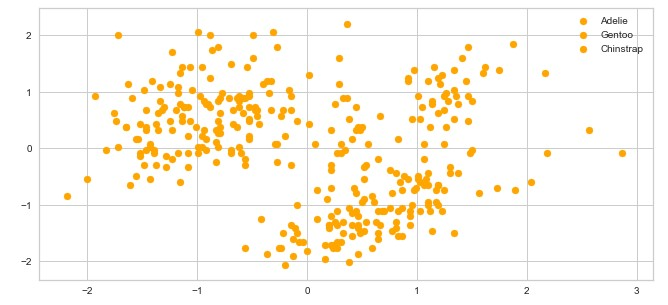How many clusters do you see? We could say 3-ish, but we can not be sure. Plus there is that data in the middle which is super sketchy. As we said one of the most popular methods for determining the number of clusters in the dataset is called the Elbow Method. It can be built on top of two metrics: distortion and inertia. Distortion is calculated as the average of the squared distances (let’s say Euclidean distance) from the cluster centers of the respective clusters. Inertia represents the sum of squared distances of samples to their closest cluster center.What we can do is run our clustering algorithm with a variable number of clusters and calculate distortion and inertia. Then we can plot the results. There we can look for the “elbow” point. This is the point after which the distortion/inertia starts decreasing in a linear fashion as the number of clusters grows. This point tells us the optimal number of clusters.

That is exactly what we did with our solution, so when we calculate the distortion and inertia for the results above and plot the distortion value along with the number of clusters: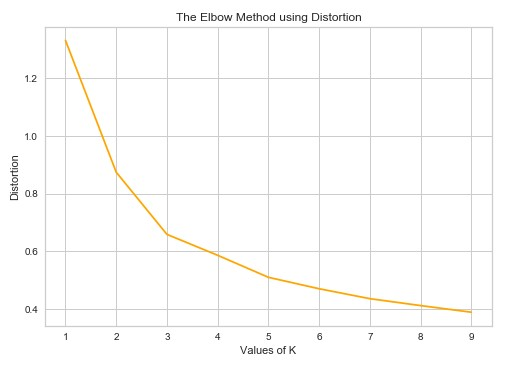From this image we can conclude that after 3 clusters, distortion decreases in a linear fashion, ie. 3 is the optimal number of clusters. How about inertia:We can conclude the same thing from this image as well.

## Conclusion

In this article, we had a chance to explore how we can utilize unsupervised learning for clustering problems. We observed the K-Means Clustering algorithm and implemented it with ML.NET. We also briefly explored algorithms like Hierarchical Clustering and DBSCAN. We applied K-Means Clustering to the PalmerPenguins dataset and saw some really interesting results. Also, we had a chance to see how powerful unsupervised learning can be.#### Nikola M. Zivkovic

CAIO at Rubik's Code

Nikola M. Zivkovic a CAIO at Rubik’s Code and the author of book “Deep Learning for Programmers“. He is loves knowledge sharing, and he is experienced speaker. You can find him speaking at meetups, conferences and as a guest lecturer at the University of Novi Sad.

Rubik’s Code is a boutique data science and software service company with more than 10 years of experience in Machine Learning, Artificial Intelligence & Software development. Check out the services we provide.

Ultimate Guide to Machine Learning with Python

Everything from Python basics to the deployment of Machine Learning algorithms to production in one place.

Become a Machine Learning Superhero TODAY!